Courses

# Current Electricity NAT Level - 1

## 10 Questions MCQ Test Topic wise Tests for IIT JAM Physics | Current Electricity NAT Level - 1

Description
This mock test of Current Electricity NAT Level - 1 for Physics helps you for every Physics entrance exam. This contains 10 Multiple Choice Questions for Physics Current Electricity NAT Level - 1 (mcq) to study with solutions a complete question bank. The solved questions answers in this Current Electricity NAT Level - 1 quiz give you a good mix of easy questions and tough questions. Physics students definitely take this Current Electricity NAT Level - 1 exercise for a better result in the exam. You can find other Current Electricity NAT Level - 1 extra questions, long questions & short questions for Physics on EduRev as well by searching above.
*Answer can only contain numeric values
QUESTION: 1

### The potential difference (in Volts) between point A and B is :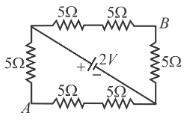Solution: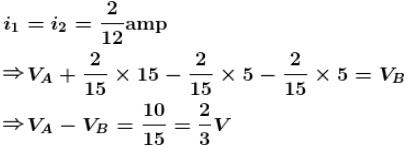*Answer can only contain numeric values
QUESTION: 2

### The total power dissipated (in Watts) in the circuit shown here is :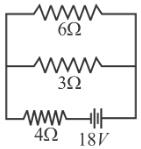Solution:

The resistances of 6Ω and 3Ω are in parallel in the given circuit, their equivalent resistance is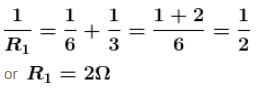Again  R1  is in series with 4Ω resistance, hence
R = R1 + 4 = 2 + 4
= 6Ω
Thus, the total power dissipated in the circuit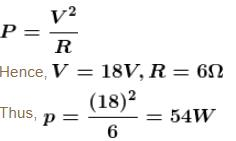*Answer can only contain numeric values
QUESTION: 3

### In the figure the potential difference across 6Ω resistor is 48V. Then the potential difference (in Volts) between A  and B  is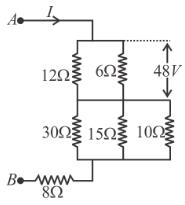Solution: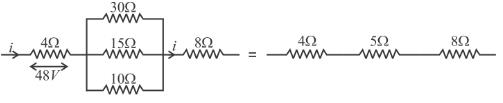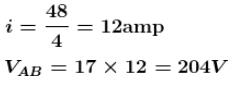*Answer can only contain numeric values
QUESTION: 4

When the resistance of copper wire is 0.1Ω and the radius is 1 mm, then the length of the wire (in meter) is (Specific resistance of copper is 3.14 × 10–8 Ωm)

Solution: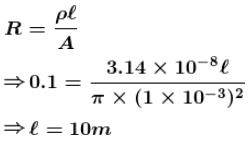*Answer can only contain numeric values
QUESTION: 5

One filament takes 10 minutes to heat a kettle and another takes 15 min. If connected in parallel they combindly take ........... minutes to heat the same kettle.

Solution:

For first filament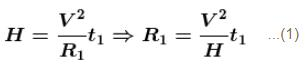For second filament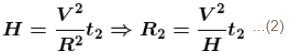When placed in parallel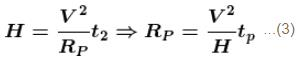From Eqs(1), (2), (3) we get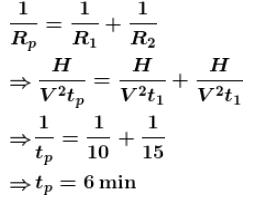*Answer can only contain numeric values
QUESTION: 6

For what value of unknown resistance X  (in Ω), the potential difference between B and D  will be zero in the arrangement of figure shown.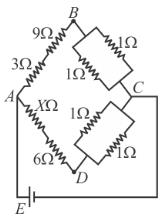Solution:

For balance bridge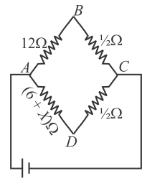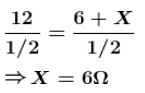*Answer can only contain numeric values
QUESTION: 7

Three resistance PQR  each of 2Ω and an unknown resistance S  from for four arms of a Wheatstone’s bridge circuit. When a resistance of 6Ω is connected in parallel to S  the bridge get balanced. What is the value of S  (in Ω)?

Solution:

The bridge formed with given resistance is a balanced wheat stone’s bridge.

The situation can be depicted as shown in figure.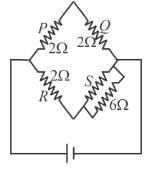As resistances S  and 6Ω are in parallel their effective resistance is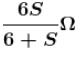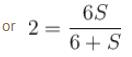or  4S = 12
or  S = 3Ω

*Answer can only contain numeric values
QUESTION: 8

For the network of resistance shown in the figure the equivalent resistance of the network between the points A  and  is 18 ohm. The value of unknown resistance  R(Ω) is :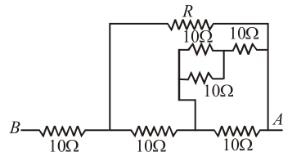Solution: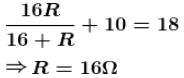*Answer can only contain numeric values
QUESTION: 9

In the circuit of figure A1 and A2 are ideal ammeters. Then the reading in ammeter A2 (in Ampere) is :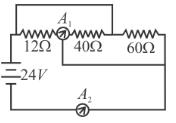Solution: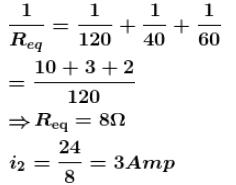*Answer can only contain numeric values
QUESTION: 10

The value of current ( in Ampere)in the circuit will be.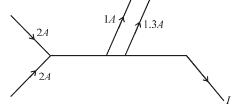Solution:

According to Kirchhoff’s junction rule,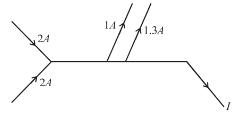Sum of incoming currents = Sum of out going currents
⇒  2 + 2 = 1 + 1.3 + I
∴  I = 1.7A